## Info

Publication number
JP6331195B2
JP6331195B2 JP2014198226A JP2014198226A JP6331195B2 JP 6331195 B2 JP6331195 B2 JP 6331195B2 JP 2014198226 A JP2014198226 A JP 2014198226A JP 2014198226 A JP2014198226 A JP 2014198226A JP 6331195 B2 JP6331195 B2 JP 6331195B2
Authority
JP
Japan
Prior art keywords
transmission beam
transmission
unit
period
Prior art date
Legal status (The legal status is an assumption and is not a legal conclusion. Google has not performed a legal analysis and makes no representation as to the accuracy of the status listed.)
Active
Application number
JP2014198226A
Other languages
Japanese (ja)
Other versions
JP2016070733A (en
Inventor

Original Assignee
パナソニックＩｐマネジメント株式会社
Priority date (The priority date is an assumption and is not a legal conclusion. Google has not performed a legal analysis and makes no representation as to the accuracy of the date listed.)
Filing date
Publication date
Application filed by パナソニックＩｐマネジメント株式会社 filed Critical パナソニックＩｐマネジメント株式会社
Priority to JP2014198226A priority Critical patent/JP6331195B2/en
Publication of JP2016070733A publication Critical patent/JP2016070733A/en
Application granted granted Critical
Publication of JP6331195B2 publication Critical patent/JP6331195B2/en
Application status is Active legal-status Critical
Anticipated expiration legal-status Critical

## Images

•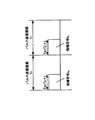•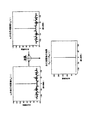•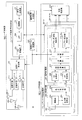•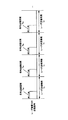•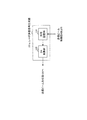•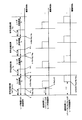•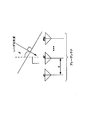•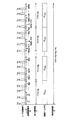•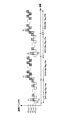•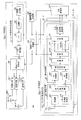•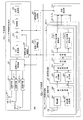•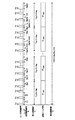•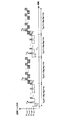•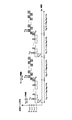•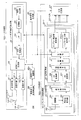•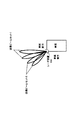•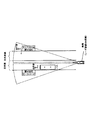•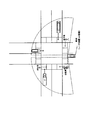•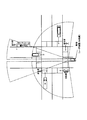•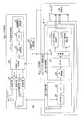•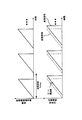## Classifications

• GPHYSICS
• G01MEASURING; TESTING
• G01S13/00Systems using the reflection or reradiation of radio waves, e.g. radar systems; Analogous systems using reflection or reradiation of waves whose nature or wavelength is irrelevant or unspecified
• G01S13/02Systems using reflection of radio waves, e.g. primary radar systems; Analogous systems
• G01S13/50Systems of measurement based on relative movement of target
• G01S13/52Discriminating between fixed and moving objects or between objects moving at different speeds
• G01S13/522Discriminating between fixed and moving objects or between objects moving at different speeds using transmissions of interrupted pulse modulated waves
• G01S13/524Discriminating between fixed and moving objects or between objects moving at different speeds using transmissions of interrupted pulse modulated waves based upon the phase or frequency shift resulting from movement of objects, with reference to the transmitted signals, e.g. coherent MTI
• G01S13/53Discriminating between fixed and moving objects or between objects moving at different speeds using transmissions of interrupted pulse modulated waves based upon the phase or frequency shift resulting from movement of objects, with reference to the transmitted signals, e.g. coherent MTI performing filtering on a single spectral line and associated with one or more range gates with a phase detector or a frequency mixer to extract the Doppler information, e.g. pulse Doppler radar
• GPHYSICS
• G01MEASURING; TESTING
• G01S13/00Systems using the reflection or reradiation of radio waves, e.g. radar systems; Analogous systems using reflection or reradiation of waves whose nature or wavelength is irrelevant or unspecified
• G01S13/02Systems using reflection of radio waves, e.g. primary radar systems; Analogous systems
• G01S13/06Systems determining position data of a target
• G01S13/08Systems for measuring distance only
• G01S13/10Systems for measuring distance only using transmission of interrupted pulse modulated waves
• G01S13/26Systems for measuring distance only using transmission of interrupted pulse modulated waves wherein the transmitted pulses use a frequency- or phase-modulated carrier wave
• G01S13/28Systems for measuring distance only using transmission of interrupted pulse modulated waves wherein the transmitted pulses use a frequency- or phase-modulated carrier wave with time compression of received pulses
• G01S13/284Systems for measuring distance only using transmission of interrupted pulse modulated waves wherein the transmitted pulses use a frequency- or phase-modulated carrier wave with time compression of received pulses using coded pulses
• GPHYSICS
• G01MEASURING; TESTING
• G01S13/00Systems using the reflection or reradiation of radio waves, e.g. radar systems; Analogous systems using reflection or reradiation of waves whose nature or wavelength is irrelevant or unspecified
• G01S13/02Systems using reflection of radio waves, e.g. primary radar systems; Analogous systems
• G01S13/0209Systems with very large relative bandwidth, i.e. larger than 10 %, e.g. baseband, pulse, carrier-free, ultrawideband
• GPHYSICS
• G01MEASURING; TESTING
• G01S13/00Systems using the reflection or reradiation of radio waves, e.g. radar systems; Analogous systems using reflection or reradiation of waves whose nature or wavelength is irrelevant or unspecified
• G01S13/02Systems using reflection of radio waves, e.g. primary radar systems; Analogous systems
• G01S13/06Systems determining position data of a target
• G01S13/08Systems for measuring distance only
• G01S13/10Systems for measuring distance only using transmission of interrupted pulse modulated waves
• G01S13/26Systems for measuring distance only using transmission of interrupted pulse modulated waves wherein the transmitted pulses use a frequency- or phase-modulated carrier wave
• G01S13/28Systems for measuring distance only using transmission of interrupted pulse modulated waves wherein the transmitted pulses use a frequency- or phase-modulated carrier wave with time compression of received pulses
• G01S13/282Systems for measuring distance only using transmission of interrupted pulse modulated waves wherein the transmitted pulses use a frequency- or phase-modulated carrier wave with time compression of received pulses using a frequency modulated carrier wave
• GPHYSICS
• G01MEASURING; TESTING
• G01S13/00Systems using the reflection or reradiation of radio waves, e.g. radar systems; Analogous systems using reflection or reradiation of waves whose nature or wavelength is irrelevant or unspecified
• G01S13/02Systems using reflection of radio waves, e.g. primary radar systems; Analogous systems
• G01S13/06Systems determining position data of a target
• G01S13/42Simultaneous measurement of distance and other co-ordinates
• GPHYSICS
• G01MEASURING; TESTING
• G01S13/00Systems using the reflection or reradiation of radio waves, e.g. radar systems; Analogous systems using reflection or reradiation of waves whose nature or wavelength is irrelevant or unspecified
• G01S13/02Systems using reflection of radio waves, e.g. primary radar systems; Analogous systems
• G01S13/50Systems of measurement based on relative movement of target
• G01S13/52Discriminating between fixed and moving objects or between objects moving at different speeds
• G01S13/522Discriminating between fixed and moving objects or between objects moving at different speeds using transmissions of interrupted pulse modulated waves
• G01S13/524Discriminating between fixed and moving objects or between objects moving at different speeds using transmissions of interrupted pulse modulated waves based upon the phase or frequency shift resulting from movement of objects, with reference to the transmitted signals, e.g. coherent MTI
• GPHYSICS
• G01MEASURING; TESTING
• G01S13/00Systems using the reflection or reradiation of radio waves, e.g. radar systems; Analogous systems using reflection or reradiation of waves whose nature or wavelength is irrelevant or unspecified
• G01S13/02Systems using reflection of radio waves, e.g. primary radar systems; Analogous systems
• G01S13/50Systems of measurement based on relative movement of target
• G01S13/58Velocity or trajectory determination systems; Sense-of-movement determination systems
• G01S13/581Velocity or trajectory determination systems; Sense-of-movement determination systems using transmission of interrupted pulse modulated waves and based upon the Doppler effect resulting from movement of targets
• G01S13/582Velocity or trajectory determination systems; Sense-of-movement determination systems using transmission of interrupted pulse modulated waves and based upon the Doppler effect resulting from movement of targets adapted for simultaneous range and velocity measurements
• GPHYSICS
• G01MEASURING; TESTING
• G01S13/00Systems using the reflection or reradiation of radio waves, e.g. radar systems; Analogous systems using reflection or reradiation of waves whose nature or wavelength is irrelevant or unspecified
• G01S13/93Radar or analogous systems specially adapted for specific applications for anti-collision purposes
• G01S13/931Radar or analogous systems specially adapted for specific applications for anti-collision purposes of land vehicles
• GPHYSICS
• G01MEASURING; TESTING
• G01S7/00Details of systems according to groups G01S13/00, G01S15/00, G01S17/00
• G01S7/02Details of systems according to groups G01S13/00, G01S15/00, G01S17/00 of systems according to group G01S13/00
• G01S7/28Details of pulse systems
• G01S7/282Transmitters
• GPHYSICS
• G01MEASURING; TESTING
• G01S7/00Details of systems according to groups G01S13/00, G01S15/00, G01S17/00
• G01S7/02Details of systems according to groups G01S13/00, G01S15/00, G01S17/00 of systems according to group G01S13/00
• G01S7/28Details of pulse systems
• GPHYSICS
• G01MEASURING; TESTING
• G01S7/00Details of systems according to groups G01S13/00, G01S15/00, G01S17/00
• G01S7/02Details of systems according to groups G01S13/00, G01S15/00, G01S17/00 of systems according to group G01S13/00
• G01S7/28Details of pulse systems
• G01S2007/2883Coherent receivers using FFT processing
• GPHYSICS
• G01MEASURING; TESTING
• G01S7/00Details of systems according to groups G01S13/00, G01S15/00, G01S17/00
• G01S7/02Details of systems according to groups G01S13/00, G01S15/00, G01S17/00 of systems according to group G01S13/00
• G01S7/28Details of pulse systems
• G01S2007/2886Coherent receivers using I/Q processing

## Description

The present disclosure relates to a radar apparatus.

In recent years, a radar apparatus using a radar transmission signal having a short wavelength including a microwave or a millimeter wave that can obtain a high resolution has been studied. In addition, in order to improve outdoor safety, development of a radar device that detects not only a vehicle but also an object (target) including a pedestrian in a wide angle range is required.

For example, a pulse radar device that repeatedly transmits a pulse wave is known as a radar device. The received signal of the wide-angle pulse radar that detects the vehicle / pedestrian in the wide-angle range is a mixture of a plurality of reflected waves from a target (for example, a vehicle) that exists at a short distance and a target (for example, a pedestrian) that exists at a long distance. Signal. Therefore, (1) the radar transmitter is required to have a configuration for transmitting a pulse wave or a pulse-modulated wave having an autocorrelation characteristic (hereinafter referred to as a low range side lobe characteristic) that has a low range side lobe, and (2) The radar receiver is required to have a wide reception dynamic range.

As a radar apparatus using a pulse wave (or pulse modulated wave) for obtaining a low range side lobe characteristic, for example, a pulse compression radar apparatus using a Barker code, an M-sequence code, a complementary code, or the like is known. As an example, a case where complementary codes are used will be described. Complementary code has two code sequences (hereinafter, .n = 1 and complementary code sequence a n, b n, ..., L.L the code sequence length) made of. The autocorrelation calculation of each of the two code sequences is expressed by the following equation.

As shown in FIG. 1, two complementary codes a n and b n are transmitted in a time division manner as complementary codes. As shown in the following equation, the complementary code is obtained by adding the results of autocorrelation calculation of two code sequences with the shift time (delay time) τ matched, thereby obtaining a correlation value with a range side lobe of 0. (See FIG. 2, for example).

A method for generating a complementary code is disclosed in Non-Patent Document 1. According to Non-Patent Document 1, for example, based on a code sequence of a = [1 1] and b = [1 −1] having complementarity composed of elements “1” or “−1”, the code sequence length L = 4, 8, 16, 32, ..., 2 P complementary codes can be generated sequentially. As the code sequence length of the complementary code is longer, the dynamic range required for reception (required reception dynamic range) is expanded. On the other hand, the shorter the code sequence length of the complementary code, the lower the peak sidelobe ratio (PSR), so even if multiple reflected waves from a short distance target and a long distance target are mixed, The required reception dynamic range can be reduced.

On the other hand, when an M-sequence code is used instead of a complementary code, the peak sidelobe ratio is given by 20 log (1 / L) [dB]. Therefore, in order to obtain a low-range side lobe in an M-sequence code, a code sequence length L longer than that of a complementary code is required (for example, L = 1024 when PSR = 60 dB).

Further, as a wide-angle radar device that detects a target in a wide-angle range, a configuration in which a radar wave is transmitted by mechanically or electronically scanning a directional beam has been proposed (see, for example, Patent Document 1). In Patent Document 1, the radar apparatus performs reception processing by switching the antenna beam direction for each predetermined antenna beam rotation stop section.

In addition, it is known that addition processing and Fourier transform processing are used in order to improve SNR (Signal to Noise Ratio) in radar reception processing of a pulse compression radar apparatus.

Specifically, when the pulse compression code is repeatedly transmitted in the pulse transmission cycle Tr, the radar receiver adds (coherent integration) the correlation value calculated by the pulse compression processing, thereby adding gain (coherent addition gain). ) Is obtained. For example, among the correlation values calculated by the pulse compression process, by performing Np additions (added for each I component and Q component of the correlation value) in a pulse transmission period with high temporal correlation, SNR is improved by Np times.

The radar receiver performs a Fourier transform process using the Nc coherent addition results, thereby detecting a frequency component (hereinafter referred to as a peak Doppler spectrum) on the Doppler spectrum, thereby coherent addition. Gain is obtained. For example, when the Doppler spectrum can be approximated to a line spectrum, the SNR is improved by Nc times. For example, FFT (Fast Fourier Transform) or DFT (Discrete Fourier Transform) may be used as the Fourier transform.

That is, the SNR is improved by (Np × Nc) times by performing addition processing and Fourier transform processing in the radar receiver.

JP 2001-228243 A

Budisin, S.Z., "New complementary pairs of sequences," Electron. Lett., 1990, 26, (13), pp.881-883

In the above-described prior art, for example, a radar apparatus that switches the transmission beam direction of a radar transmission signal at predetermined intervals by beam scanning in a radar transmission unit as in Patent Document 1, and performs addition processing and Fourier transform processing in a radar reception unit Is assumed to be used. In such a radar apparatus, radar reception processing is performed for each transmission beam direction. However, when a target moving at high speed is a detection target, it is required to shorten the beam scanning time. Thus, for example, reduction of the number of additions in each of the coherent integration process and the Fourier transform process in the radar reception process can be considered.

On the other hand, as described above, the radar apparatus is required to have high resolution. Here, the Doppler frequency for the number of additions (sometimes called a coherent addition number) Np in the addition process (coherent integration process) and the number of additions (sometimes called a Doppler addition number) Nc in the Fourier transform process. The resolution Δfd and the maximum observable Doppler frequency fd_max are expressed by the following equations.
Δfd = 1 / (Np × Nc × Tr) (3)
fd_max = ± 1 / (2Np × Tr) (4)

As shown in the above equations (3) and (4), in order to shorten the beam scanning time, when the coherent addition number Np for each transmission beam is decreased, the Doppler frequency resolution Δfd is decreased and the maximum Doppler frequency fd_max is increased. . If the Doppler addition number Nc for each transmission beam is reduced in order to shorten the beam scanning time, the Doppler frequency resolution Δfd is lowered and the maximum Doppler frequency fd_max is maintained.

As described above, the conventional technique has a problem that the beam scanning time cannot be shortened while maintaining the Doppler frequency resolution.

An object of one aspect of the present disclosure is to provide a radar apparatus that can shorten the beam scanning time while maintaining the Doppler frequency resolution.

A radar apparatus according to an aspect of the present disclosure transmits a radar signal at a predetermined transmission period, and performs a coherent integration process and a Doppler frequency analysis process on a reflected wave signal obtained by reflecting the radar signal to a target A transmission beam control unit that switches a transmission beam direction used for transmitting the radar signal from a plurality of transmission beam directions, and the switched transmission beam direction among the plurality of transmission beam directions. A radar transmission unit for transmitting the radar signal, wherein the plurality of transmission beam directions are grouped into a plurality of transmission beam sets each including at least two transmission beam directions, and the transmission beam control unit includes: The plurality of transmissions in a period of a first period in which the Doppler frequency analysis processing is performed on one transmission beam set Sequentially switching the Musetto sequentially switches the at least two transmit beam directions contained in said one transmit beams within the first period.

According to one aspect of the present disclosure, it is possible to shorten the beam scanning time while maintaining the Doppler frequency resolution.

Hereinafter, embodiments according to one aspect of the present disclosure will be described in detail with reference to the drawings. In the embodiment, the same components are denoted by the same reference numerals, and the description thereof will be omitted because it is duplicated.

FIG. 3 is a block diagram showing a configuration of the radar apparatus 10 according to the present embodiment.

The radar apparatus 10 includes a radar transmitter 100, a radar receiver 200, a reference signal generator 300, and a transmission beam controller 400.

The radar transmitter 100 generates a high-frequency radar signal (radar transmission signal) based on the reference signal received from the reference signal generator 300. Then, the radar transmitter 100 switches the transmission beam direction according to the control of the transmission beam controller 400 and transmits a radar transmission signal at a predetermined transmission cycle.

The reference signal generation unit 300 is connected to each of the radar transmission unit 100 and the radar reception unit 200. The reference signal generator 300 supplies a reference signal as a reference signal to the radar transmitter 100 and the radar receiver 200 in common, and synchronizes the processing of the radar transmitter 100 and the radar receiver 200.

The transmission beam control unit 400 controls the main beam direction θ TX of the transmission beam when transmitting a radar transmission signal. That is, the transmission beam control unit 400 switches the transmission beam direction used for transmitting the radar transmission signal from a plurality of settable transmission beam directions.

Specifically, a plurality of configurable transmit beam directions are grouped into a plurality of “transmit beam sets” each including at least two transmit beam directions. Here, the number of transmit beam directions contained in each transmit beams is referred to as "transmit beams beam number 'represents the N BS. Then, the transmission beam control unit 400 performs control so that the transmission beam directions included in the transmission beam set are sequentially switched every predetermined first switching period T1. In addition, the transmission beam control unit 400 sequentially switches the transmission beam set for each predetermined second switching period T2 that is an integer multiple of the first switching period T1.

Then, the transmission beam control unit 400 indicates switching of the main beam direction to the radar transmission unit 100 (transmission beam forming unit 106 described later) and the radar reception unit 200 (switching unit 212 and azimuth estimation unit 214 described later). Output a control signal. The detailed operation of the transmission beam control in the transmission beam control unit 400 will be described later.

The radar transmission unit 100 includes a radar transmission signal generation unit 101, a transmission beam forming unit 106, transmission RF units 107-1 to 107-N_Tx, and transmission antennas 108-1 to 108-N_Tx. That is, the radar transmission unit 100 includes N_Tx transmission RF units 107 and transmission antennas 108, respectively.

The radar transmission signal generation unit 101 generates a timing clock obtained by multiplying the reference signal received from the reference signal generation unit 300 by a predetermined number, and generates a radar transmission signal based on the generated timing clock. The radar transmission signal generation unit 101 repeatedly outputs a radar transmission signal at a predetermined radar transmission cycle (Tr). The radar transmission signal is represented by r (n, M) = I (k, M) + jQ (k, M). Here, j represents an imaginary unit, k represents a discrete time, and M represents an ordinal number of the radar transmission period.

The radar transmission signal generation unit 101 includes a code generation unit 102, a modulation unit 103, an LPF (Low Pass Filter) 104, and a DA conversion unit 105.

Specifically, the code generation unit 102, for each radar transmission period Tr, code a n code sequence with a code length L (n = 1, ..., L) to produce a (pulse compression code). Examples of the code sequence include an M-sequence code, a Barker code sequence, a complementary code sequence (including a Golay code sequence, a Spano code sequence, and the like).

For example, when using a complementary code sequence as the code sequence, the code generation unit 102 generates code Pn in the pair alternately radar transmission every cycle, Qn (a n shown in FIG. 1, corresponding to b n), respectively. That is, the code generation unit 102 outputs one code Pn constituting a pair of complementary codes to the modulation unit 103 as a code in the M-th radar transmission cycle (represented as Tr [M]), and continues to the (M In the (+1) th radar transmission cycle (represented as Tr [M + 1]), the other code Qn constituting the pair of complementary codes is output to the modulator 103 as a code. Similarly, in the (M + 2) th and subsequent radar transmission cycles, the code generation unit 102 uses the Mth and (M + 1) th radar transmission cycles as one unit to generate a code Pn. , Qn are repeatedly generated and output to the modulation unit 103.

Modulator 103, a pulse modulation to the code a n received from the code generation unit 102 (amplitude modulation, ASK (Amplitude Shift Keying), pulse shift keying) is performed or a phase modulation (Phase Shift Keying), a modulated signal to LPF104 Output.

The LPF 104 outputs only a signal component equal to or lower than a predetermined limit band from the modulation signal received from the modulation unit 103 to the DA conversion unit 105 as a baseband radar transmission signal. Note that the LPF unit 104 may be arranged at a subsequent stage of the DA conversion unit 105 described later.

The DA conversion unit 105 converts the digital transmission signal received from the LPF 104 into an analog transmission signal, and outputs the analog transmission signal to the transmission beam forming unit 106.

The transmission beam forming unit 106 forms a transmission beam in the main beam direction θ Tx (u) according to an instruction from the transmission beam control unit 400 with respect to the baseband radar transmission signal received from the radar transmission signal generation unit 101. Specifically, the transmission beam forming unit 106 sets the transmission beam direction θ TX (u) instructed from the transmission beam control unit 400 to the multiple N_Tx transmission RF units 107 and the transmission antennas 108. The radar transmission signal is multiplied by a weighting coefficient WTx (Index_Tx, θ TX (u) ), and the multiplied signal is output to the transmission RF section 107.

For example, when the transmission antennas 108 are arranged in a straight line at equal intervals (element spacing d), the transmission beam forming unit 106 corrects the amplitude / phase deviation between the transmission antennas 108, and then the weighting coefficient represented by the following equation: By using WTx (Index_Tx, θTx (u) ), the transmission beam direction is variably formed.

Here, Index_Tx = 1,..., N_Tx, λ is the wavelength of the radar transmission signal (transmission RF signal), and d is the element spacing of the transmission antenna 108.

When the main beam is directed in the direction θ Tx (u) and the sidelobe level is further reduced, the transmission beam forming unit 106 is configured to use a weighting coefficient (for example, a Dorf-Chebyshev) composed of a phase component and an amplitude component. Beam weight, Taylor beam weight, etc.) may be used.

The Index_Tx (= 1,..., N_Tx) -th transmission RF unit 107 performs frequency conversion on the transmission signal multiplied by the weighting coefficient WTx (Index_Tx, θ Tx (u) ) and performs carrier frequency (Radio Frequency: RF). ) Band radar transmission signal is generated, amplified to a predetermined transmission power P [dB] by a transmission amplifier, and output to the transmission antenna 108.

The Index_Tx (= 1,..., N_Tx) th transmission antenna 108 radiates the radar transmission signal received from the Index_Tx (= 1,..., N_Tx) th transmission RF unit 107 into space.

FIG. 4 shows a radar transmission signal transmitted from the radar transmitter 100. The code transmission section Tw includes a pulse code sequence having a code length L. In each radar transmission cycle Tr, a pulse code sequence is transmitted during the code transmission section Tw, and the remaining section (Tr-Tw) is a no-signal section. By performing pulse modulation using No samples per pulse code (a n ), a signal of Nr (= No × L) samples is included in each code transmission section Tw. . That is, the sampling rate in the modulation unit 103 is (No × L) / Tw. Further, it is assumed that Nu samples are included in the no-signal section (Tr-Tw).

The radar transmitter 100 may include a radar transmission signal generator 101a shown in FIG. 5 instead of the radar transmission signal generator 101. The radar transmission signal generation unit 101a does not include the code generation unit 102, the modulation unit 103, and the LPF 104 illustrated in FIG. 3, but includes a code storage unit 110 instead. The code storage unit 110 stores in advance the code sequence generated by the code generation unit 102 (FIG. 3), and reads the code sequence according to the control signal from the transmission beam control unit 400.

In FIG. 3, the radar receiver 200 includes N_Rx reception antennas 202, and configures an array antenna. The radar receiver 200 includes N_Rx antenna system processors 201-1 to 201-N_Rx and a direction estimator 214.

Each antenna system processing unit 201 includes a reception antenna 202, a reception RF unit 203, and a signal processing unit 207.

The reception antenna 202 receives a reflected wave signal that is a radar transmission signal reflected by the target (object), and outputs the received reflected wave signal to the reception RF unit 203 as a reception signal.

The reception RF unit 203 includes an amplification unit 204, a frequency converter 205, and a quadrature detector 206. The reception RF unit 203 generates a timing clock obtained by multiplying the reference signal received from the reference signal generation unit 300 by a predetermined number, and operates based on the generated timing clock. Specifically, the amplifier 204 amplifies the received signal received from the receiving antenna 202 to a predetermined level, the frequency converter 205 converts the frequency of the received signal in the high frequency band to the baseband, and the quadrature detector 206 A band-band received signal is converted into a baseband-band received signal composed of an I signal and a Q signal.

The signal processing unit 207 includes AD conversion units 208 and 209, a correlation calculation unit 210, an addition unit 211, a switching unit 212, and a Doppler frequency analysis unit 213. The signal processing unit 207 includes a transmit beams the number of beams N BS pieces of the Doppler frequency analyzing unit 213.

The AD converter 208 receives the I signal from the quadrature detector 206, and the AD converter 209 receives the Q signal from the quadrature detector 206. The AD conversion unit 208 converts the I signal into digital data by sampling the baseband signal composed of the I signal in discrete time. The AD conversion unit 209 converts the Q signal into digital data by performing sampling in discrete time on the baseband signal including the Q signal.

Here, in the sampling of the AD conversion units 208 and 209, Ns discrete samples are performed per time Tp (= Tw / L) of one pulse in the radar transmission signal. That is, the number of oversamples per pulse is Ns.

In the following description, the discrete time of the Mth radar transmission cycle Tr [M] as the output of the AD converters 208 and 209 using the I signal Ir (k, M) and the Q signal Qr (k, M). The baseband received signal at k is expressed as complex signal x (k, M) = Ir (k, M) + jQr (k, M). In the following description, the discrete time k is a sampling point before the end of the radar transmission cycle Tr. The signal processing unit 207 uses the timing at which the radar transmission cycle (Tr) starts as a reference (k = 1). = Operates periodically up to (Nr + Nu) Ns / No. That is, k = 1,..., (Nr + Nu) Ns / No.

Correlation calculation section 210, for each radar transmission period Tr, discrete sample values x (k, M) consisting of discrete sample values Ir (k, M) and Qr (k, M) received from AD conversion sections 208 and 209, pulse compression codes a n of the code length L to be transmitted in a radar transmitter 100 (where, n = 1, ..., L ) correlation calculation to perform. For example, correlation calculation section 210 performs a discrete sample values x (k, M), a sliding correlation operation between the pulse compression code a n. For example, the correlation calculation value AC (k, M) of the sliding correlation calculation at the discrete time k in the Mth radar transmission cycle Tr [M] is calculated based on the following equation.

In the above formula, an asterisk (*) represents a complex conjugate operator.

The correlation calculation unit 210 performs the correlation calculation over a period of k = 1,..., (Nr + Nu) Ns / No, for example, according to Equation (6).

Note that the correlation calculation unit 210 is not limited to the case where the correlation calculation is performed on k = 1,..., (Nr + Nu) Ns / No, and the measurement is performed according to the presence range of the target to be measured by the radar apparatus 10. The range (ie, the range of k) may be limited. As a result, the radar apparatus 10 can reduce the calculation processing amount of the correlation calculation unit 210. For example, the correlation calculation unit 210 may limit the measurement range to k = Ns (L + 1),..., (Nr + Nu) Ns / No-NsL. In this case, as shown in FIG. 6, the radar apparatus 10 does not perform measurement in a time interval corresponding to the code transmission interval Tw. Thereby, even when the radar transmission signal directly wraps around the radar receiving unit 200, the radar apparatus 10 does not perform the processing by the correlation calculation unit 210 during the period during which the radar transmission signal wraps around (a period of at least less than τ1). Therefore, measurement without the influence of wraparound becomes possible. When the measurement range (range of k) is limited, the processing of the adding unit 211, the first Doppler frequency analyzing unit 213-1, the second Doppler frequency analyzing unit 213-2, and the direction estimating unit 214 described below is performed. Similarly, a process that limits the measurement range (range k) may be applied. Thereby, the processing amount in each component can be reduced, and the power consumption in the radar receiver 200 can be reduced.

The adder 211 uses the correlation calculation value AC (k, M) received from the correlation calculation unit 210 at each discrete time k of the Mth radar transmission cycle Tr, and uses a predetermined number (Np) of radar transmission cycles Tr. The correlation calculation value AC (k, M) is added (coherent integration) over a period (Tr × Np). Addition (coherent integration) processing of the addition number Np over a period (Tr × Np) is expressed by the following equation.

Here, CI (k, m) represents an addition value of correlation calculation values (hereinafter also referred to as a correlation addition value), Np is an integer value of 2 or more, and m is the number of additions Np in the addition unit 211. Is a natural number indicating the ordinal number of additions in the case where is a unit.

The addition unit 211 performs Np additions using the output of the correlation calculation unit 210 obtained in units of the radar transmission cycle Tr as one unit. That is, the adding unit 211 uses the correlation calculation values AC (k, Np (m−1) +1) to AC (k, Np × m) as one unit, and adds the correlation values CI with the timings of the discrete times k aligned. (K, m) is calculated for each discrete time k. Thereby, the addition part 211 can improve SNR of a reflected wave signal in the range in which the reflected wave signal from a target has a high correlation by the effect of addition over Np times of a correlation calculation value. Therefore, it is possible to improve the measurement performance related to the estimation of the target arrival distance.

In order to obtain an ideal addition gain, a condition is required in which the phase components of the correlation calculation value are aligned within a certain range in the addition interval of the correlation calculation value addition count Np. That is, the number of additions Np is preferably set based on the assumed maximum moving speed of the target to be measured. This is because the larger the assumed maximum speed of the target, the larger the amount of fluctuation of the Doppler frequency included in the reflected wave from the target, and the shorter the time period having high correlation. In this case, since the number of additions Np is a small value, the gain improvement effect by the addition in the adding unit 211 is reduced.

Switching unit 212 in accordance with an instruction from the transmission beam control unit 400, as the output destination of the signal received from the adder 211 for each discrete time k, N BS pieces Doppler frequency analyzing unit (corresponding to transmit beams the number of beams) 213- 1-213-N Selectively switch BS .

For example, when the transmission beam set beam number N BS = 2, two Doppler frequency analyzes 213 are provided. The Doppler frequency analysis unit 213-1 receives the (2m-1) th output CI (k, 2m-1) of the addition unit 211 obtained at each discrete time k. In addition, the (2m) -th output CI (k, 2m) of the adder 211 obtained at every discrete time k is input to the Doppler frequency analysis unit 213-2. Here, k = 1,... (Nr + Nu) Ns / No.

Further, when the number of transmission beam set beams is N BS , the signal processing unit 207 is configured to include N BS Doppler frequency analysis units 213. For example, the y-th Doppler frequency analysis unit 213-y receives the N BS (m−1) + y-th output CI (k, N BS (m− 1) + y) is entered. Here, y = 1, ..., a N BS, k = 1, ... , a (Nr + Nu) Ns / No .

The Doppler frequency analysis unit 213 performs Doppler frequency analysis processing on the output of the addition unit 211 received from the switching unit 212. Hereinafter, transmit beams the number of beams as N BS, a description will be given of the operation in the y-th Doppler frequency analyzing unit 213-y. Here, y = 1, ..., a N BS.

The Doppler frequency analysis unit 213-y outputs Nc outputs CI (k, N BS Nc (w-1) -1) + y) to CI (k, K) obtained from the adder 211 obtained at each discrete time k. N BS (Nc × w−1) + y) as a unit, and coherent integration is performed with the timing of discrete time k aligned. For example, the Doppler frequency analysis unit 213-y corrects the phase fluctuation Φ (fs) = 2πfs (Tr × Np × N BS ) ΔΦ corresponding to 2Nf different Doppler frequencies fsΔΦ as shown in the following equation. , Perform coherent integration.

Here, FT_CI y, Nant (k, fs, w) is the w-th output in the Doppler frequency analysis unit 213-y, and the Doppler frequency fsΔΦ at the discrete time k in the Nant-th antenna system processing unit 201. The coherent integration result of is shown. However, Nant = 1 to N_Rx, fs = -Nf + 1, ..., 0, ..., Nf, k = 1, ..., (Nr + Nu) Ns / No, w is a natural number, and ΔΦ is Phase rotation unit.

Thereby, each antenna system processing unit 201 performs FT_CIy, Nant (k, −Nf + 1, w),... FT_CIy, Nant (coherent integration results corresponding to 2Nf Doppler frequency components for each discrete time k. k, Nf−1, w) is obtained for a plurality of Np × N BS × Nc periods (Tr × Np × N BS × Nc) of the radar transmission cycle Tr, that is, every second switching cycle. J is an imaginary unit.

When ΔΦ = 1 / Nc, the above-described processing of the Doppler frequency analysis unit 213 performs discrete Fourier transform on the output of the addition unit 211 at the sampling interval Tm = (Tr × Np × N BS ) and the sampling frequency fm = 1 / Tm. (DFT) Equivalent to processing.

Further, by setting Nf to a power of two, the Doppler frequency analysis unit 213 can apply a fast Fourier transform (FFT) process, and can greatly reduce the amount of calculation processing. At this time, when Nf> Nc, by performing zero padding processing with CI (k, N BS (Nc (w-1) + q) + y) = 0 in a region where q> Nc, Similarly, FFT processing can be applied, and the amount of calculation processing can be greatly reduced.

Further, the Doppler frequency analysis unit 213 may perform a process of sequentially calculating the product-sum operation shown in the above equation (8) without performing the FFT process. That is, the Doppler frequency analysis unit 213 calculates Nc outputs of CI (k, N BS (Nc (w−1) + q) + y) of the addition unit 211 obtained at each discrete time k. Coefficients exp [-j2πfsTrNpNBSqΔφ] corresponding to fs = −Nf + 1,..., 0,. Here, q = 0 to Nc-1.

In the following description, the output FT_CI y, 1 (from the y-th Doppler frequency analysis unit 213-y obtained by performing the same processing in each of the Na (= N_Rx) antenna system processing units 201 k, fs, w), FT_CI y, 2 (k, fs, w), ..., FT_CI y, Na (k, fs, w) are combined into a correlation vector h y (k, fs, w) write. The correlation vector h y (k, fs, w) is used to describe processing for estimating the direction based on the phase difference between the receiving antennas 202 with respect to the reflected wave signal from the target, which will be described later. Here, y = 1, ..., a N BS.

Further, instead of the correlation matrix h y (k, fs, w) shown in Equation (9), a correlation vector is calculated using one of the plurality of antenna system processing units 201 as a reference phase as shown in the following equation. May be.

Here, the superscript asterisk (*) indicates a complex conjugate operator. Also, k = 1,... (Nr + Nu) Ns / No.

The processing in each component of the signal processing unit 207 has been described above.

The direction estimation unit 214 performs an array correction value on the correlation vector h y (k, fs, w) output from the w th Doppler frequency analysis unit 213-y output from the antenna system processing units 201-1 to 201-N_Rx. Is used to calculate the correlation vector hy_after_cal (k, fs, w) in which the phase deviation and amplitude deviation between the antenna system processing units 201 are corrected. The correlation vector h y_after_cal (k, fs, w) is expressed by the following equation.

Then, the direction estimation unit 214 performs the direction estimation process based on the phase difference of the reflected wave signal between the reception antennas 202 using the correlation vector hy_after_cal (k, fs, w). Specifically, the direction estimation unit 214 performs the discrete time k, the Doppler frequency fsΔΦ, or the discrete time k when the norm of h y _after_cal (k, fs, w) or its square value is equal to or greater than a predetermined value, Using the correlation vector h y _ after_cal (k, fs, w) corrected for phase deviation and amplitude deviation for the Doppler frequency fsΔΦ, the azimuth direction θ u shown in the following equation is variable, and the direction estimation evaluation function value P yu , k, fs, w) is calculated.

Here, y = 1, ..., a N BS, u = 1, ... , a NU (number of configurable transmission beam direction). Note that arg max P (x) is an operator whose output value is the value of the domain where the function value P (x) is maximum. The azimuth estimation unit 214 sets the azimuth direction θ u in which the maximum value of the direction estimation evaluation function values P yu , k, fs, w) is obtained as the arrival direction estimation value DOA y (k, fs, w).

Note that the direction estimation unit 214 may perform a process of limiting the variable range of the azimuth direction θ u during the direction estimation process based on the control signal received from the transmission beam control unit 400. That is, when the correlation vector h y (k, fs, w) from the w th Doppler frequency analysis unit 213-y is output for the main beam direction θ Tx of the transmission beam, The variable range of the azimuth direction θ u may be limited to θ Tx −BW / 2 ≦ θ Tx ≦ θ Tx + BW / 2. Here, the BW may use an angle about the beam width of the transmission beam. As a result, the range of direction estimation processing is limited to about the beam width in the main beam direction of the transmission beam, and the reception SNR of the reflected wave from the target existing in the transmission beam direction is improved, so that the accuracy of arrival angle estimation is improved. Can do. Furthermore, it is possible to suppress the reflected wave from the target existing at an angle separated by about the beam width in the transmission beam direction. Therefore, it is possible to suppress the deterioration of the arrival angle separation performance even when reflected waves having the number N_Rx or more of the receiving antennas 202 exist in the same distance bin and the same Doppler bin.

There are various evaluation function values P yu , k, fs, w) depending on the arrival direction estimation algorithm. For example, an estimation method using an array antenna disclosed in Reference Non-Patent Document 1 may be used.

(Reference Non-Patent Document 1) Direction-of-arrival estimation using signal subspace modeling Cadzow, J.A .; Aerospace and Electronic Systems, IEEE Transactions on Volume: 28, Issue: 1 Publication Year: 1992, Page (s): 64-79

For example, the beamformer method can be expressed as: Other methods such as Capon and MUSIC are also applicable.

Here, the superscript H is a Hermitian transpose operator. H y_after_cal (k, fs, w) is a correlation matrix, and any one of the following equations (14) to (17) may be used. In Expressions (15) to (17), DL is a predetermined integer value.

Here, noise is suppressed by generating a correlation matrix including discrete times (B = (k−DL) to (k + DL)) adjacent to the discrete time k as shown in Expression (15). As a result, the accuracy of the direction estimation process can be improved. Moreover, noise is suppressed by generating a correlation matrix including a Doppler frequency component (B = (fs-DL) to (fs + DL)) adjacent to the Doppler frequency fsΔΦ as shown in Expression (16). As a result, the accuracy of the direction estimation process can be improved. Moreover, noise can be suppressed by generating a correlation matrix including outputs before and after the w-th output (B = (w−DL) to (w + DL)) as shown in Expression (17). As a result, the accuracy of the direction estimation process can be improved.

The direction estimation unit 214, the w-th arrival direction estimate DOA y calculated (k, fs, w) based on the discrete time k, the Doppler frequency fsΔΦ and angle theta u, output as radar positioning result To do.

Here, an N_Rx-th order column vector is defined as a direction vector a (θ u ) with the complex response of the array antenna (receiving antenna 202) when a reflected wave with respect to the radar transmission signal arrives from the θ u direction. The direction vector a (θ u ) represents a phase difference calculated geometrically by the element spacing between the receiving antennas 202. For example, when array antennas (receiving antennas 202) are arranged on a straight line at equal intervals d as shown in FIG. 7, the direction vector a (θ u ) is expressed by the following equation.

Here, θ u is obtained by changing the azimuth range in which the arrival direction is estimated at a predetermined azimuth interval β. For example, θ u is set as follows.
θ u = θmin + uβ, u = 0,…, NU
NU = floor [(θmax-θmin) / β] +1
Here, floor (x) is a function that returns the maximum integer value not exceeding the real number x.

The time information k described above may be converted into distance information and output. When converting the time information k into the distance information R (k), the following equation may be used. Here, Tw represents the code transmission interval, L represents the pulse code length, and C 0 represents the speed of light.

The Doppler frequency information (fsΔΦ) may be converted into a relative velocity component and output. When converting the Doppler frequency fsΔΦ to the relative velocity component vd (fs), the following equation may be used. Here, λ is the wavelength of the carrier frequency of the RF signal output from the transmission RF unit 107.

The operation of the radar apparatus 10 having the above configuration will be described.

The transmission beam control operation in the transmission beam control unit 400 will be described with reference to FIGS. 8 and 9.

The transmission beam control unit 400 performs variable control in the main beam direction at intervals of Δθ Tx within the beam variable range θ min ≦ θ Tx ≦ θ max .

Here, the transmission beam control unit 400 transmits the transmission beam direction (mainly used for transmission of the radar transmission signal ) from among the four transmission beam directions θ Tx (u1) to θ Tx ( u4 ) in the beam variable range. Set the beam direction. Here, a transmission beam set including (θ Tx (u1) , θ Tx (u2) ) and a transmission beam set including (θ Tx (u3) , θ Tx (u4) ) are used. That is, the transmission beam set beam number NBS is 2. The transmission beam set number may be three or more, transmit beams the number of beams N BS may be three or more.

(1) As illustrated in FIG. 8, the transmission beam control unit 400 sequentially switches a plurality of transmission beam directions included in the transmission beam set for each first switching period T1. For example, in FIG. 8, the transmission beam control unit 400 switches the transmission beam direction θ Tx (u1) and θ Tx (u2) in order for each first switching period T1.

As shown in FIG. 8, the period of the first switching period T1 (corresponding to the second period) is, for example, the product of the radar transmission period Tr and the addition number Np of the correlation calculation value (that is, the reflected wave signal) in the coherent integration process. Represented by That is, in FIG. 8, the period of the first switching period T1 corresponds to a period during which coherent integration processing in which the reflected wave signal (correlation calculation value) is added Np times in the radar receiver 200 is performed.

The transmission beam control unit 400 repeats the operation (1) within a period (corresponding to the first period) of the second switching period T2 that is an integer multiple of the first switching period T1. For example, in FIG. 8, the second switching period T2 is expressed as N BS Nc times (here, N BS = 2) the first switching period T1. In other words, the period of the second switching period T2 is a period of the first switching period T1 (Tr × Np), and transmit beams the number of beams N BS, coherent integration result (correlation sum value in the processing of the Doppler frequency analyzing unit 213 ) And the addition number Nc. That is, in FIG. 8, the period of the second switching period T2, in the radar receiver 200, a period in which the Doppler frequency analysis processing is performed on the coherent addition results in N BS pieces of transmission beam direction.

(2) As shown in FIG. 9, the transmission beam control unit 400 switches the transmission beam set for each second switching period T2. For example, in FIG. 9, the transmission beam control unit 400 transmits a transmission beam set (θ Tx (u1) , θ Tx (u2) ) and a transmission beam set (θ Tx (u3) , θ for each second switching period T2. Tx (u4) )

The transmission beam control unit 400 repeats the operation (2) until the transmission of radar transmission signals using all the transmission beam sets (two in FIG. 9) is completed.

(3) The transmission beam control unit 400 repeats the operations (1) and (2).

The radar transmission unit 100 (transmission beam forming unit 106) sets the transmission beam direction set by the transmission beam control unit 400 from the transmission beam directions that scan the beam variable range at predetermined angular intervals. That is, the radar transmitter 100 transmits a radar transmission signal by switching the transmission beam direction in units of addition numbers (first switching period T1) for coherent integration in the radar receiver 200 (adder 211). Further, the radar transmitter 100 transmits a radar transmission signal by switching the transmission beam set at the second switching period.

When switching the transmission beam, a certain amount of time (transition time T Beam_SW ) may be required until the switching operation is stabilized due to the characteristics of the analog circuit that performs transmission beam formation in the transmission beam forming unit 106. In such a case, the transmission beam control unit 400 has a first switching period T1 and a second switching period at a time timing (for example, an early timing at least before the transition time T Beam_SW ) before the timing of the code transmission interval. T2 may be sent out. Thereby, even in the code transmission section immediately after the transmission beam switching, the transmission operation of the analog circuit that performs the transmission beam formation in the transmission beam forming unit 106 is completed, and the radar transmission unit 100 transmits the pulse code string in a stable state. be able to.

On the other hand, the radar receiver 200 (adder 211) passes the correlation calculation value AC (k, M) over the first switching period T1 (= Tr × Np) for each discrete time k, for example, according to the equation (7). (That is, Np times) is added to calculate the correlation addition value CI (k, m). For example, in FIG. 8, an adder 211, for each first switching period T1, the transmission beam direction theta correlation sum values for Tx (u1) CI (k, m), and the correlation with respect to the transmission beam direction θ Tx (u2) The addition value CI (k, m) is calculated.

Then, the radar receiver 200 (switching unit 212), the discrete time correlation sum value obtained for each k CI (k, M), and transmit beams the number of beams N Doppler frequency analysis unit provided in correspondence to the BS Switch to 213-1 to 213-N BS and output. For example, in FIG. 8, the switching unit 212 outputs the (2m−1) -th output CI (k, 2m−1) from the adding unit 211 to the Doppler frequency analyzing unit 213-1 and The (2m) -th output CI (k, 2m) is output to the Doppler frequency analysis unit 213-2. Here, m is an ordinal number incremented every first switching period T1, with the first first switching period T1 shown in FIG.

That is, the switching unit 212 outputs the correlation addition values CI (k, m) for the transmission beam directions θ Tx (u1) and θ Tx (u2) shown in FIG. 8 to different Doppler frequency analysis units 213, respectively. As a result, as shown in FIG. 8, within the second switching period T2, Nc correlation addition values CI (k, m) for the transmission beam direction θ Tx (u1) are given to the Doppler frequency analysis unit 213-1. Then, Nc correlation addition values CI (k, m) with respect to the transmission beam direction θ Tx (u2) are input to the Doppler frequency analysis unit 213-2.

Then, the radar receiver 200 (each Doppler frequency analyzer 213) calculates the equation (8 ) for Nc correlation addition values CI (k, M) with respect to the transmission beam direction θ Tx (u1) or θ Tx (u2) . ) To detect the peak Doppler spectrum by performing Doppler frequency analysis.

As described above, the radar apparatus 10 according to the present embodiment has 1 within the period of the second switching period T2 in which Doppler frequency analysis processing is performed on transmission beam sets each including at least two transmission beam directions. The transmission beam directions included in one transmission beam set are sequentially switched. Moreover, the radar apparatus 10 switches a several transmission beam set in order for every 2nd switching period T2. Further, the radar apparatus 10 sequentially switches the direction of the transmission beam included in the transmission beam set for each first switching period T1 of the period for performing the coherent integration process within the period of the second switching period T2.

Thereby, the observation time of the Doppler frequency in the radar apparatus 10 with respect to each transmission beam direction included in the transmission beam set is Np × Nc × N BS × Tr. That is, as shown in FIG. 8, by alternately switching the transmission beam direction in the transmission beam set, the period (setting time) for setting each transmission beam direction is Np × Nc × Tr. observation time transmit beam direction of the Doppler frequency becomes N BS times the set time. In this way, when viewed in the direction of one transmission beam included in the transmission beam set, the time interval for outputting the coherent integration result can be made longer than the addition time interval for performing the coherent integration.

For example, as compared with a case where only a single transmission beam direction (for example, θ Tx (u1) ) is set in an observation time (for example, T2) of a predetermined Doppler frequency, this embodiment includes the transmission beam set. The period during which one transmitted beam direction (for example, θ Tx (u1) ) is set is 1 / N BS . That is, in this embodiment, when viewed around one transmission beam direction included in the transmission beam set, coherent while maintaining the Doppler frequency observation time when only a single transmission beam direction is set. The number of integration additions (addition time) can be shortened.

For example, as shown in Equation (3), the Doppler frequency resolution Δfd depends on the Doppler frequency observation time (Np × Nc × Tr). According to the present embodiment, even if the coherent addition number Np is reduced to 1 / N BS (the addition time is shortened), the Doppler frequency observation time is (Np × N BS × Nc × Tr), and the coherent It remains the same as before the number of additions decreased. That is, even if the number of coherent additions per transmission beam direction is reduced, deterioration of Doppler frequency resolution when performing Doppler frequency analysis can be suppressed.

In this way, even if the addition time interval for performing the coherent integration in the adding unit 211 is shortened by increasing the number of transmission beam directions included in the transmission beam set, the time interval (Doppler) for outputting the coherent integration result is reduced. Frequency observation time) can be made as long as before the addition time interval for performing coherent integration in the addition unit 211 is shortened. Therefore, according to the present embodiment, it is possible to shorten the beam scanning time while maintaining the Doppler frequency resolution.

Further, according to the present embodiment, since the addition time interval for performing coherent integration per transmission beam direction can be shortened, reduction of coherent addition gain due to coherent integration in addition unit 211 is suppressed even when the target moves at high speed. be able to.

(Modification 1)
In this embodiment, instead of providing a plurality of Doppler frequency analyzing unit 213 in accordance with the transmit beams the number of beams N BS as shown in FIG. 3, as shown in FIG. 10, according to transmit beams the number of beams N BS A plurality of buffers 220 may be provided. Specifically, in the radar apparatus 10a shown in FIG. 10, the buffer 220-1～ buffer 220-N BS correspond respectively to each transmit beam direction of the transmit beams.

Switching unit 212a is a correlation sum value CI for each transmission beam direction (k, M), and outputs switch to corresponding buffers 220-1 to 220-N BS. That is, the buffer 220 temporarily stores the correlation addition value CI (k, M) for the corresponding transmission beam direction.

Similar to the switching unit 212a, the switching unit 221 switches the output of the correlation addition value CI (k, M) from the buffer 220 corresponding to each transmission beam direction according to the control of the transmission beam control unit 400. That is, the switching unit 221 serially outputs the correlation addition value CI (k, M) for each transmission beam to the Doppler frequency analysis unit 213a in units of time intervals (Np × Nc × Tr).

The Doppler frequency analysis unit 213a performs Doppler frequency analysis processing on the correlation addition value CI (k, M) for each transmission beam input serially.

Also in the radar apparatus 10a having such a configuration, the beam scanning time can be shortened while maintaining the Doppler frequency resolution as described above.

(Modification 2)
In the second modification, a case will be described in which a radar transmission signal that periodically fluctuates is generated using a radar transmission period longer than the first switching period T1, which is a period for switching each transmission beam direction of the transmission beam set.

FIG. 11 is a block diagram illustrating a configuration of a radar apparatus 10c according to the second modification.

Based on the control of the transmission beam control unit 400, the code generation control unit 120 generates a radar transmission signal generation unit that generates a radar transmission signal that maintains a desired periodicity for each transmission beam direction included in the transmission beam set. 101 is controlled. In other words, the code generation control unit 120 transmits the codes of one code sequence that cannot be transmitted at a time within one period of the first switching period T1 in order over a plurality of periods. Control for each transmission beam direction.

Specifically, the code generation control unit 120, based on the first switching cycle T1 and the second switching cycle T2 instructed from the transmission beam control unit 400, the radar transmission signal for each transmission beam direction included in the transmission beam set. Count the transmission cycle. That is, the code generation control unit 120, based on the first switching period T1, for each transmit beams the number of beams N BS, i.e., increments the count value of the radar transmission cycle for each transmission beam direction. Moreover, the code generation control unit 120 resets the count value of the radar transmission period for each transmission beam direction based on the second switching period T2.

The radar transmission signal generation unit 101b performs the above operation for each transmission beam direction included in the transmission beam set.

In this way, for example, as shown in FIG. 8, even when the transmission beam direction is switched every first switching period T1 within the second switching period T2, the radar transmission signal generation unit 101b is provided for each transmission beam direction. A radar transmission signal maintaining a desired periodicity can be generated.

Therefore, even when a radar transmission signal that periodically varies using a radar transmission period longer than the first switching period T1 is used, the beam scanning time is shortened while maintaining the Doppler frequency resolution, as in the above embodiment. be able to.

(Modification 3)
In the above embodiment, the transmission beam control unit 400 sets the first switching period T1 to the same value for each transmission beam direction included in the transmission beam set, as shown in FIGS. The case where the correlation calculation value included in the first switching period T1 having the same value is added for each transmission beam direction has been described.

On the other hand, in Modification 3, a case will be described in which the periods of the first switching period T1 in which each of a plurality of transmission beam directions included in the transmission beam set is set are different from each other. Specifically, the transmission beam control unit 400 transmits transmission included in the beam set Beamset beam number N with respect to BS, different length the first switching cycle of T1 (1), T1 (2 ), ..., T1 (NBS) is used to switch the transmission beam direction.

An example of the operation of the modification 3 will be described with reference to FIGS. 12 and 13. In FIG. 12 and FIG. 13, similar to FIG. 8 and FIG. 9, in the beam variable range, from among the four transmit beam direction θ Tx (u1) ~θ Tx ( u4), used for transmission of the radar transmission signal The main beam direction is set. Two transmission beam sets (θ Tx (u1) , θ Tx (u2) ) and (θ Tx (u3) , θ Tx (u4) ) are set. That is, in FIG. 12 and FIG. 13, N BS = 2.

As shown in FIG. 13, for the two transmission beam directions (θ Tx (u1), θ Tx (u2) ) in one transmission beam set, the transmission beam control unit 400 performs first switching with different lengths. The transmission beam is switched using the periods T1 (1) and T1 (2) . Similarly, as shown in FIG. 13, the transmission beam control unit 400 performs different first switching for the two transmission beam directions (θ Tx (u3) and θ Tx (u4) ) in one transmission beam set. The transmission beam is switched using the periods T1 (3) and T1 (4) .

In FIG. 12, the addition unit 211 performs Np one- time radar transmission signal (correlation calculation value) addition processing for each transmission switching direction θ Tx (u1) for each first switching period T1 (1). . Similarly, in FIG. 12, the adding unit 211 performs Np two times of radar transmission signal (correlation calculation value) addition processing for each transmission switching direction θ Tx (u2) for each first switching period T1 (2). Do. The same applies to the transmission beam directions θ Tx (u3) and θ Tx (u4) . That is, the addition unit 211, N BS pieces of transmission beam direction (θ Tx (u1), θ Tx (u2), ..., θ Tx (uBS)) with respect to the first switching period corresponding to each T1 (1 ), T1 (2), ... , T1 ( addition number Np 1 contained BS2), Np 2, ..., performs addition processing at Np BS.

Thus, in the third modification, the switching period can be made variable for each transmission beam direction included in the transmission beam set. In the addition unit 211, addition processing is performed with the number of additions included in the first switching period T1. Therefore, by making the first switching cycle T1 variable for each transmission beam direction, the addition gain by coherent addition in the adder 211 can be set variably.

For example, when a planar antenna is configured by the transmission antenna 108, a transmission beam in the front direction of the planar antenna can obtain a higher directivity gain than a transmission beam in an oblique direction. Accordingly, when one transmission beam set includes transmission beam directions having different directivity gains, the transmission beam control unit 400 sets the first switching period T1 for the transmission beam direction having a high directivity gain to the directivity gain. May be set shorter than the first switching period T1 in the direction of the transmission beam having a low value. That is, it is set longer as the period of the first switching period T1 with respect to the transmission beam direction having a low directivity gain among the transmission beam directions included in the transmission beam set.

By doing so, the coherent addition gain (that is, the number of additions) for the transmission beam direction with a low directivity gain is higher than the coherent addition gain for the transmission beam direction with a high directivity gain. That is, in Modification 3, the detection distance in the detection area (each transmission beam direction) of the radar apparatus 10 can be smoothed in consideration of both the directivity gain and the coherent addition gain.

Note that the transmission beam control unit 400 may set the first switching period T1 to be longer with respect to the transmission beam direction in which the detection distance needs to be extended in the detection area in the transmission beam set. By doing so, the detection distance in the detection area can be adjusted to a desired value.

For example, when the radar apparatus 10 is mounted on the front side of the vehicle, a detection distance is required in the transmission beam direction corresponding to the vehicle front direction than in the transmission beam direction corresponding to the vehicle side surface direction. Therefore, the transmission beam control unit 400 may set the first switching cycle T1 for the transmission beam direction corresponding to the vehicle front direction longer than the first switching cycle for the transmission beam direction corresponding to the vehicle side surface direction. By doing so, it is possible to extend the detection distance in the vehicle front direction.

Further, the transmission beam control unit 400 may perform control so that the sum of the first switching periods T1 with respect to each transmission beam direction in the transmission beam set is the same among the plurality of transmission beam sets. For example, in FIG. 14, the transmission beam sets (θ Tx (u1) , θ Tx (u2) ) are set with the first switching periods T1 (1) , T1 (2) , and the transmission beam sets (θ Tx (u3) , θ Tx (u4) ) is set with the first switching periods T1 (3) and T1 (4) . In this case, the transmission beam control unit 400 sets the first switching period T1 for each transmission beam direction so that T1 (1) + T1 (2) = T1 (3) + T1 (4) .

Here, in the period using the transmission beam set (θ Tx (u1) , θ Tx (u2) ), the adding unit 211 has N BS = 2 transmission beam directions (θ Tx (u1), θ Tx (u2 ). ) ), Addition processing is performed with the addition numbers Np 1 and Np 2 included in the first switching periods T1 (1) and T1 (2) , respectively. Similarly, in the period using the transmission beam set (θ Tx (u3) , θ Tx (u4) ), the adding unit 211 has N BS = 2 transmission beam directions (θ Tx (u3), θ Tx (u4)). ) Is added using the addition numbers Np 3 and Np 4 included in the first switching periods T1 (3) and T1 (4) , respectively.

From the relationship T1 (1) + T1 (2) = T1 (3) + T1 (4) , the sampling interval of the output from the adder 211 that is the input of each Doppler frequency analysis unit 213 is the same between each transmission beam set. It becomes. Therefore, the Doppler frequencies fsΔΦ (where fs = −Nf + 1,..., 0,..., Nf) are the same, and the Doppler frequency resolution is the same for all the transmission beams. As a result, an effect is obtained in which an interpolation process for matching Doppler frequencies for each transmission beam included in different transmission beam sets is unnecessary.

(Modification 4)
In the fourth modification, a case where the radar apparatus according to the present disclosure is mounted on a moving body such as a vehicle will be described.

FIG. 15 is a block diagram illustrating a configuration of a radar apparatus 10d according to the fourth modification. The radar apparatus 10d is different from the radar apparatus 10 shown in FIG. 3 in that a vehicle traveling state detection unit 500 is added and the transmission beam control unit 400a operates based on an output from the vehicle traveling state detection unit 500.

For example, the vehicle traveling state detection unit 500 detects the vehicle speed based on sensor information received from a vehicle speed sensor (not shown) of the vehicle on which the radar device 10d is mounted, and outputs the detection result to the transmission beam control unit 400a. .

The transmission beam control unit 400a performs transmission beam control based on the detection result received from the vehicle travel state detection unit 500. Specifically, the transmission beam control unit 400a may set the transmit beams the number of beams N BS according to the vehicle speed. For example, the transmit beam controller 400a, the transmit beams the number of beams N BS when the vehicle speed is equal to or less than a predetermined speed L speed, from transmit beams the number of beams N BS when the vehicle speed exceeds a predetermined speed L speed May also be increased. For example, when L speed = 40 km / h, the transmission beam control unit 400a determines that when the vehicle traveling speed exceeds 40 km / h, the transmission beam set beam number N BS = 2 and the vehicle traveling speed is 40 km / h or less. N BS = 3 may be set.

In addition, the transmission beam control unit 400a may increase the first switching period T1 (= Tr × Np) when the vehicle speed is equal to or lower than the predetermined speed L speed .

Here, the second switching period T2 is represented by (Tr × Np × N BS × N C ). Therefore, when the vehicle running speed is less than L speed, since transmit beams the number of beams N BS increases, the second switching period T2 becomes longer. Therefore, in the Doppler frequency analysis processing in the Doppler frequency analysis unit 213, the sampling interval becomes longer and the Doppler frequency observation time becomes longer, so that the Doppler frequency resolution is improved.

On the other hand, when the vehicle speed falls below the predetermined speed L speed , the maximum observable Doppler frequency fd_max is reduced. However, in situations where the vehicle traveling speed is less than or equal to a predetermined value, the assumed maximum Doppler frequency will be small, and even if the maximum observable Doppler frequency fd_max is small, it will prevent erroneous detection of the Doppler frequency. Can do.

As described above, in Modification 4, transmission beam control unit 400a, based on the running state of the vehicle which the radar device 10d is mounted, for controlling the transmit beams the number of beams N BS. Specifically, when the vehicle traveling speed is equal to or lower than a predetermined value, the assumed maximum Doppler frequency is reduced, so that processing with improved resolution of the Doppler frequency component in the Doppler frequency analysis unit 213 is possible. Therefore, according to the radar apparatus 10d, the detection performance of an object that moves at a low speed, such as a pedestrian, can be improved. Or the detection performance of the object which moves to the cross range direction orthogonal to the distance range direction of the radar apparatus 10d can also be improved.

When the radar apparatus 10d is mounted in front of the vehicle, the transmission beam control unit 400a may perform the following process according to the detection result of the vehicle traveling state detection unit 500.

Specifically, the transmission beam control unit 400a is based on the output of the vehicle traveling state detection unit 500 when the vehicle speed exceeds the predetermined speed L speed as compared with the case where the vehicle speed is equal to or less than the predetermined speed L speed. The number of transmission beam sets to be used is decreased and the first switching period T1 is lengthened. As shown in FIG. 16, the transmission beam set is composed of a transmission beam set 1 in which the front side of the vehicle is the transmission beam direction and a transmission beam set 2 in which the side of the vehicle is the transmission beam direction.

For example, when the vehicle traveling speed is equal to or lower than a predetermined speed L speed (60 km / h), the transmission beam control unit 400a transmits the transmission beam set 1 (θ Tx (u1), θ Tx (u2 ) with the front of the vehicle as the transmission beam direction. ), ...) And the transmission beam set 2 (θ Tx (u3), θ Tx (u4) ) with the side of the vehicle as the transmission beam direction are alternately used.

On the other hand, when the vehicle traveling speed exceeds a predetermined speed L speed (60 km / h), the transmission beam control unit 400a transmits only the transmission beam set 1 (θ Tx (u1), θ Tx (u2), ...). use. Furthermore, the transmission beam control unit 400a lengthens the first switching period T1 (for example, about twice).

As described above, in the vehicle in which the radar apparatus 10d is mounted in front of the vehicle, the transmission beam control unit 400a makes the number of transmission beam sets to be used variable based on the vehicle traveling state. Furthermore, the transmission beam control unit 400a lengthens the first switching period T1 when the number of transmission beam sets is reduced.

As a result, when the vehicle traveling speed exceeds a predetermined value, the first switching cycle T1 can be lengthened using only the transmission beam set whose forward beam direction is the front of the vehicle. The detection distance can be made longer. Therefore, the radar apparatus 10d can confirm the safety in the vehicle front direction earlier, and can enhance the preventive safety effect as the in-vehicle radar.

For example, main traffic accidents when a vehicle travels on a single road include a rear-end collision with a preceding vehicle, a collision with a road structure, or a collision with a road crossing person (including jumping out). In order to prevent or reduce such traffic accidents, the radar apparatus 10d needs to detect objects and people present on the lane or on the sidewalk.

FIG. 17 shows an example of a detection target and a detection range when the vehicle on which the radar device 10d is mounted is traveling straight on a single road. Among the detection targets, a vehicle (a bus in FIG. 17), which is a representative moving object, exists on the roadway, and a bicycle or a pedestrian exists on the sidewalk or roadway.

Further, in general, the vehicle speed during single road traveling is higher than the vehicle speed when traveling near an intersection. For this reason, in order to prevent a traffic accident, the radar apparatus 10d is required to detect earlier in time, that is, to detect a longer distance. For example, when traveling at 60 km / h, the radar device 10d can detect a target 16.6 m / s x 3 seconds = about 50 m in order to complete detection of the detection target 3 seconds before the collision prediction time. It is necessary to become.

On the other hand, as for the angular direction, as shown in FIG. 17, it is only necessary to cover the own lane and the opposite lane as a roadway and a sidewalk parallel to the roadway. Since the lane width of ordinary roads in Japan is 3.5m, for example, the total width is about 15m for 2 lanes on one side and 4 lanes including oncoming lanes. Therefore, during traveling on a single road, the radar device 10d has a viewing angle of about 30 degrees on the left and right (total of about 60 degrees) with the traveling direction of the host vehicle being 0 degrees, and the detection range is sufficient.

On the other hand, in the vicinity of the intersection as shown in FIG. 18, not only the own lane and the oncoming lane, but also a road crossing the own lane and a pedestrian crossing where bicycles or pedestrians pass are also detected by the radar device 10 d. There is a need to. For this reason, as shown in FIG. 18, the radar device 10 d requires a wider detection range than when traveling on a single road (FIG. 17). However, since the vehicle speed at the intersection is generally low, the detection distance by the radar device 10d may be shorter than that during traveling on a single road (FIG. 17).

As shown in FIGS. 17 and 18, the radar apparatus 10 d can switch to a long-distance narrow angle during high-speed traveling (for example, during single-road traveling) and a short-distance wide angle during low-speed traveling (when entering an intersection). Good. By doing so, the radar apparatus 10d can effectively switch the detection range according to the situation of the vehicle on which the radar apparatus 10d is mounted or the surrounding situation, and as a result, traffic accidents can be reduced and prevented. It is possible to contribute.

As shown in FIG. 19, the radar apparatus 10d may switch the detection range according to the detection direction. In FIG. 19, a long-distance narrow-angle detection range is set on the front side of the vehicle on which the radar apparatus 10 d is mounted, and a short-distance wide-angle detection range is set on the side side.

(Modification 5)
In the above embodiment, the case where the pulse compression radar is used has been described. In the fifth modification, a radar system using a frequency-modulated pulse wave such as a chirp pulse radar will be described.

FIG. 20 is a block diagram illustrating a configuration of a radar apparatus 10e according to the fifth modification. The radar device 10e uses chirp pulses in the radar transmitter 100.

In the radar transmitter 100, the modulation signal generator 130 periodically generates a sawtooth-shaped frequency-modulated modulation signal shown in FIG. Here, the transmission cycle of the modulated signal is T chirp .

A VCO (Voltage Controlled Oscillator) 131 outputs a frequency modulation signal based on a signal frequency-modulated by a modulation signal received from the modulation signal generator 130.

The DA conversion unit 132 converts the digital transmission signal received from the VCO 131 into an analog transmission signal, and outputs the analog transmission signal to the transmission beam forming unit 106.

Here, the radar transmission signal generation unit 101c variably controls the transmission cycle T chirp so as to coincide with the first switching cycle T1 based on the first switching cycle T1 instructed from the transmission beam control unit 400.

The directional coupling unit 133 outputs some signals radiated from the plurality of transmission antennas 108 to the mixer unit 230 of the radar receiving unit 200. Other signals are radiated from the transmitting antenna 108 to the space as radio waves.

In the radar receiving unit 200, the mixer unit 230 mixes the reflected wave signal of the radar transmission signal with the transmission signal received from the directional coupling unit 133.

The LPF 231 extracts a beat signal having a frequency (beat frequency) corresponding to the delay time of the reflected wave signal by allowing only the signal component of the reception signal after mixing to pass a predetermined limit band or less. For example, as shown in FIG. 21, a beat signal having a beat frequency is obtained from a reflected wave signal (received signal).

In the signal processing unit 207, the AD conversion unit 232 performs AD conversion on the beat signal and converts it to digital data.

The FFT unit 233 performs an FFT process on the sample data (N data ) in the transmission cycle T chirp unit, whereby a frequency spectrum in which a beat frequency peak corresponding to the delay time of the reflected wave signal appears (beat frequency spectrum). Response). Here, the beat frequency spectrum response obtained by the m-th chirp pulse transmission period is represented as CI_chirp (fb, m). Here, fb is an FFT bin number, and fb = 1,..., N data / 2.

Switching unit 234 in accordance with a control signal from the transmission beam control unit 400, the output of the FFT unit 233, selectively switches as inputs to the number of Doppler frequency analyzing unit 213 which corresponds to the transmit beams the number of beams N BS.

For example, when the transmission beam set beam number N BS = 2, two Doppler frequency analyzes 213 are provided. The Doppler frequency analysis unit 213-1 receives the (2m-1) th output CI_chirp (fb, 2m-1) of the FFT unit 233. Further, the (2m) -th output CI_chirp (fb, 2m) of the FFT unit 233 is input to the Doppler frequency analysis unit 213-2.

Further, when the number of transmission beam set beams is N BS , the signal processing unit 207 is configured to include N BS Doppler frequency analysis units 213. For example, the first y-th Doppler frequency analyzing unit 213-y, the N BS of the FFT unit 233 (m-1) + y th output CI_chirp (fb, N BS (m -1) + y) is input The Here, y = 1, ..., a N BS, fb = 1, ... , a N data / 2.

Hereinafter, transmit beams the number of beams as N BS, a description will be given of the operation in the y-th Doppler frequency analyzing unit 213-y. Here, y = 1, ..., a N BS.

The Doppler frequency analysis unit 213-y outputs Nc outputs of the FFT unit 223 obtained for each beat frequency fb, CI_chirp (fb, N BS Nc (w−1) + y) to CI_chirp (fb, N BS ( Using Nc × w-1) + y) as a unit, coherent integration is performed using the beat frequency fb. For example, the Doppler frequency analysis unit 213-y corrects the phase variation Φ (fs) = 2πfs (Tchirp × N BS ) ΔΦ according to 2Nf different Doppler frequencies fsΔΦ as shown in the following equation, and then coherently. Perform integration.

Here, FT_CI y, Nant (fb, fs, w) is the w-th output in the Doppler frequency analysis unit 213-y, and the Doppler frequency fsΔΦ at the beat frequency fb in the Nant-th antenna system processing unit 201 The coherent integration result of is shown. However, a Nant = 1~N_Rx, fs = -Nf + 1, ..., 0, ..., is Nf, fb = 1, ..., a N data / 2, w is a natural number, ΔΦ is the phase rotation Unit.

Thus, the antenna system processing unit 201, a coherent integration result corresponding to 2Nf number of Doppler frequency components for each beat frequency fb FT_CIy, Nant (fb, -Nf + 1, w), ..., FT_CIy, Nant ( fb, Nf−1, w) are obtained for a plurality of N BS × Nc periods (Tchirp × N BS × Nc) of the radar transmission cycle Tchirp. J is an imaginary unit.

With the above configuration and operation, even when a frequency-modulated pulse wave such as a chirp pulse radar is used, the beam scanning time can be shortened while maintaining the Doppler frequency resolution as in the above embodiment. .

The embodiments according to one aspect of the present disclosure have been described above.

In addition, you may implement combining the operation | movement which concerns on the said embodiment and each modification suitably.

In the radar apparatus 10 shown in FIG. 3, the radar transmitter 100 and the radar receiver 200 may be individually arranged at physically separated locations.

Although not shown, the radar apparatus 10 includes, for example, a CPU (Central Processing Unit), a storage medium such as a ROM (Read Only Memory) that stores a control program, and a working memory such as a RAM (Random Access Memory). . In this case, the function of each unit described above is realized by the CPU executing the control program. However, the hardware configuration of the radar apparatus 10 is not limited to this example. For example, each functional unit of the radar apparatus 10 may be realized as an IC (Integrated Circuit) that is an integrated circuit. Each functional unit may be individually made into one chip, or may be made into one chip so as to include a part or all thereof.

<Summary of this disclosure>
The radar apparatus according to the present disclosure is a radar apparatus that transmits a radar signal at a predetermined transmission cycle, and performs a coherent integration process and a Doppler frequency analysis process on a reflected wave signal reflected from the target. A transmission beam control unit that switches a transmission beam direction used for transmission of the radar signal from among a plurality of transmission beam directions; and the radar signal in the switched transmission beam direction among the plurality of transmission beam directions. A plurality of transmission beam directions, each of the plurality of transmission beam directions being grouped into a plurality of transmission beam sets each including at least two transmission beam directions; The plurality of transmission beam sets in a period of a first period in which the Doppler frequency analysis processing is performed on a beam set Sequentially switching, in order to switch the at least two transmit beam directions contained in said one transmit beams within the first period.

In the radar apparatus according to the present disclosure, the transmission beam control unit sequentially switches the at least two transmission beam directions in a period of a second period in which the coherent integration process is performed in the first period.

In the radar device according to the present disclosure, the second periods in which each of the at least two transmission beam directions is set are different from each other.

Moreover, in the radar apparatus according to the present disclosure, the second period with respect to the transmission beam direction having a low directivity gain among the at least two transmission beam directions is longer.

Further, in the radar device according to the present disclosure, the radar device is mounted on a vehicle, and the second period with respect to a transmission beam direction corresponding to a forward direction of the vehicle among the at least two transmission beam directions is a side surface of the vehicle. It is longer than the second period with respect to the transmission beam direction corresponding to the direction.

In the radar apparatus according to the present disclosure, the radar apparatus is mounted on a vehicle, and the transmission beam control unit calculates the number of the at least two transmission beam directions when the vehicle speed of the vehicle is a predetermined threshold value or less. More than the number of the at least two transmission beam directions when the vehicle speed exceeds the threshold.

Further, in the radar device according to the present disclosure, the radar device is mounted on a vehicle, and the transmission beam control unit compares the vehicle speed of the vehicle with a value equal to or lower than the threshold when the vehicle speed exceeds a predetermined threshold. Thus, the number of transmission beam sets to be used among the plurality of transmission beam sets is decreased, and the second period is lengthened.

In the radar apparatus according to the present disclosure, the second period is a product of a transmission period of the radar transmission signal and the number of additions of the reflected wave signal in the coherent integration process, and the first period is the second period. It is the product of the period, the number of the at least two transmission beam directions, and the addition number of the coherent integration result in the Doppler frequency analysis processing.

The present disclosure is suitable for a radar apparatus that scans a transmission beam and detects a wide-angle range, and that can suppress degradation of resolution in relative speed detection based on Doppler frequency detection and can shorten a scanning time.

10, 10a, 10c, 10d, 10e Radar apparatus 100 Radar transmitter 200 Radar receiver 300 Reference signal generator 400, 400a Transmit beam control unit 500 Vehicle traveling state detector 101, 101a, 101b, 101c Radar transmission signal generator 102 Code generator 103 Modulator 104, 231 LPF
105, 132 DA converter 106 Transmission beam forming section 107 Transmission RF section 108 Transmitting antenna 110 Code storage section 120 Code generation control section 130 Modulation signal generation section 131 VCO
133 Directional coupling unit 201 Antenna system processing unit 202 Reception antenna 203 Reception RF unit 204 Amplifier 205 Frequency converter 206 Quadrature detector 207 Signal processing unit 208, 209, 232 AD conversion unit 210 Correlation calculation unit 211 Addition unit 212, 212a, 221, 234 Switching unit 213, 213a Doppler frequency analysis unit 214 Direction estimation unit 220 Buffer 230 Mixer unit 233 FFT unit

## Claims (8)

1. A radar apparatus that transmits a radar signal at a predetermined transmission cycle and performs a coherent integration process and a Doppler frequency analysis process on a reflected wave signal reflected by the radar signal,
A transmission beam control unit that switches a transmission beam direction used for transmitting the radar signal from a plurality of transmission beam directions;
A radar transmitter that transmits the radar signal in the switched transmission beam direction among the plurality of transmission beam directions;
Comprising
The plurality of transmit beam directions are grouped into a plurality of transmit beam sets, each including at least two transmit beam directions;
The transmission beam control unit includes:
Sequentially switching the plurality of transmission beam sets in a period of a first period in which the Doppler frequency analysis processing is performed on one transmission beam set;
Sequentially switching the at least two transmit beam directions included in the one transmit beam set within the first period;
2. The transmission beam control unit includes:
Within the first period, the at least two transmission beam directions are sequentially switched in a period of a second period in which the coherent integration process is performed.
The radar apparatus according to claim 1.
3. The second periods in which each of the at least two transmit beam directions is set are different from each other;
The radar apparatus according to claim 2.
4. Of the at least two transmit beam directions, the longer the second period with respect to the transmit beam direction having a low directivity gain,
The radar apparatus according to claim 3.
5. The radar device is mounted on a vehicle,
Of the at least two transmission beam directions, the second period with respect to the transmission beam direction corresponding to the forward direction of the vehicle is longer than the second period with respect to the transmission beam direction corresponding to the lateral direction of the vehicle.
The radar apparatus according to claim 3.
6. The radar device is mounted on a vehicle,
The transmission beam control unit determines the number of the at least two transmission beam directions when the vehicle speed of the vehicle is equal to or less than a predetermined threshold, and the number of the at least two transmission beam directions when the vehicle speed of the vehicle exceeds the threshold. More than,
The radar apparatus according to claim 1.
7. The radar device is mounted on a vehicle,
The transmission beam control unit, when the vehicle speed of the vehicle exceeds a predetermined threshold, the number of transmission beam sets to be used in the plurality of transmission beam sets as compared to the case where the vehicle speed of the vehicle is equal to or less than the threshold. And lengthening the second period,
The radar apparatus according to claim 2.
8. The second period is a product of the transmission period of the radar signal and the addition number of the reflected wave signal in the coherent integration process,
The first period is a product of the second period, the number of the at least two transmission beam directions, and the addition number of the coherent integration result in the Doppler frequency analysis process.
The radar apparatus according to claim 2.
JP2014198226A 2014-09-29 2014-09-29 Radar equipment Active JP6331195B2 (en)

## Priority Applications (1)

Application Number Priority Date Filing Date Title
JP2014198226A JP6331195B2 (en) 2014-09-29 2014-09-29 Radar equipment

## Applications Claiming Priority (4)

Application Number Priority Date Filing Date Title
JP2014198226A JP6331195B2 (en) 2014-09-29 2014-09-29 Radar equipment
CN201510507573.8A CN105467381A (en) 2014-09-29 2015-08-18 Radar device
EP15183221.9A EP3001218B1 (en) 2014-09-29 2015-09-01 Radar device
US14/846,680 US10379209B2 (en) 2014-09-29 2015-09-04 Radar device

## Publications (2)

Publication Number Publication Date
JP2016070733A JP2016070733A (en) 2016-05-09
JP6331195B2 true JP6331195B2 (en) 2018-05-30

# Family

## Family Applications (1)

Application Number Title Priority Date Filing Date
JP2014198226A Active JP6331195B2 (en) 2014-09-29 2014-09-29 Radar equipment

## Country Status (4)

US (1) US10379209B2 (en)
EP (1) EP3001218B1 (en)
JP (1) JP6331195B2 (en)
CN (1) CN105467381A (en)

## Families Citing this family (5)

* Cited by examiner, † Cited by third party
Publication number Priority date Publication date Assignee Title
CN106105311B (en) * 2015-02-28 2019-11-26 华为技术有限公司 The method, apparatus and user equipment and system of a kind of data transmission
US10505643B2 (en) * 2015-03-26 2019-12-10 Lg Electronics Inc. Method and device for estimating doppler frequency by using beam scanning process in wireless communication system
US20180011181A1 (en) * 2016-07-07 2018-01-11 Infineon Technologies Ag Radar systems and methods thereof
US10455636B2 (en) * 2016-09-16 2019-10-22 Nec Corporation Link packing in mmWave networks
GB2576308A (en) * 2018-08-10 2020-02-19 Jaguar Land Rover Ltd An apparatus and method for providing driver assistance of a vehicle

## Family Cites Families (18)

* Cited by examiner, † Cited by third party
Publication number Priority date Publication date Assignee Title
JPH01153989A (en) * 1987-12-11 1989-06-16 Nec Corp Phased array radar device
JPH08274529A (en) * 1995-03-31 1996-10-18 Toshiba Corp Array antenna system
JP3639124B2 (en) * 1998-08-31 2005-04-20 株式会社東芝 Pulse radar equipment
CN100347976C (en) * 1998-09-08 2007-11-07 西门子公司 Method for forming or detecting single sequence and transmitter unit and receiver unit
JP3391284B2 (en) * 1998-12-25 2003-03-31 三菱電機株式会社 Radar equipment
JP3577239B2 (en) * 1999-05-28 2004-10-13 三菱電機株式会社 Radar equipment
JP3520829B2 (en) 2000-02-18 2004-04-19 三菱電機株式会社 Doppler radar device and beam scanning method thereof
JP2003066140A (en) 2001-08-28 2003-03-05 Murata Mfg Co Ltd Radar
JP3729127B2 (en) * 2001-12-13 2005-12-21 株式会社村田製作所 Radar
JP4375064B2 (en) * 2004-03-10 2009-12-02 株式会社デンソー Radar equipment
DE112006001358T5 (en) * 2005-06-15 2008-03-20 Murata Mfg. Co., Ltd., Nagaokakyo radar device
US20070189412A1 (en) * 2006-02-15 2007-08-16 Samsung Electronics Co., Ltd. Method and system for sounding packet exchange in wireless communication systems
JP2008145423A (en) * 2006-11-15 2008-06-26 Matsushita Electric Ind Co Ltd Radar system
JP2008275460A (en) * 2007-04-27 2008-11-13 Mitsubishi Electric Corp Radar device
US8259006B2 (en) * 2010-03-02 2012-09-04 Src Inc. Interleaved beam coherent radar apparatus and processing method
CN103918018B (en) * 2011-11-14 2017-05-10 丰田自动车株式会社 Driving assistance device
US8947647B2 (en) * 2011-12-13 2015-02-03 Raytheon Company Range-resolved vibration using large time-bandwidth product LADAR waveforms
JP6116126B2 (en) * 2012-03-29 2017-04-19 パナソニック株式会社 Radar equipment

## Also Published As

Publication number Publication date
EP3001218A2 (en) 2016-03-30
EP3001218A3 (en) 2016-04-06
US10379209B2 (en) 2019-08-13
EP3001218B1 (en) 2018-11-07
JP2016070733A (en) 2016-05-09
US20180074181A1 (en) 2018-03-15
CN105467381A (en) 2016-04-06

## Similar Documents

Publication Publication Date Title
JP6313981B2 (en) Radar apparatus, vehicle control system, and signal processing method
US20170363731A1 (en) On-demand multi-scan micro doppler for vehicle
JP6304257B2 (en) Radar apparatus, vehicle, and moving body speed detection method
JP6406601B2 (en) Radar apparatus and object detection method
US9070972B2 (en) Wideband beam forming device; wideband beam steering device and corresponding methods
US20150226848A1 (en) Method and apparatus for detecting target using radar
KR101137088B1 (en) Integrated Radar Apparatus and Integrated Antenna Apparatus
US8941533B2 (en) Method and device for detecting azimuth
JP5478010B2 (en) Electronic scanning radar equipment
US8779969B2 (en) Radar device for detecting azimuth of target
Sun et al. Analysis and comparison of MIMO radar waveforms
US7791530B2 (en) Time duplex apparatus and method for radar sensor front-ends
DE112007003175B4 (en) Electronically scanning radar system
JP5062225B2 (en) Target detection device

## Legal Events

Date Code Title Description
A621 Written request for application examination

Free format text: JAPANESE INTERMEDIATE CODE: A621

Effective date: 20170420

A977 Report on retrieval

Free format text: JAPANESE INTERMEDIATE CODE: A971007

Effective date: 20180322

TRDD Decision of grant or rejection written
A01 Written decision to grant a patent or to grant a registration (utility model)

Free format text: JAPANESE INTERMEDIATE CODE: A01

Effective date: 20180403

A61 First payment of annual fees (during grant procedure)

Free format text: JAPANESE INTERMEDIATE CODE: A61

Effective date: 20180413

R151 Written notification of patent or utility model registration

Ref document number: 6331195

Country of ref document: JP

Free format text: JAPANESE INTERMEDIATE CODE: R151